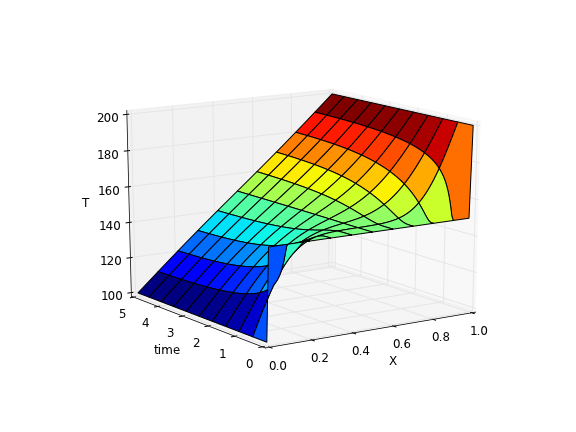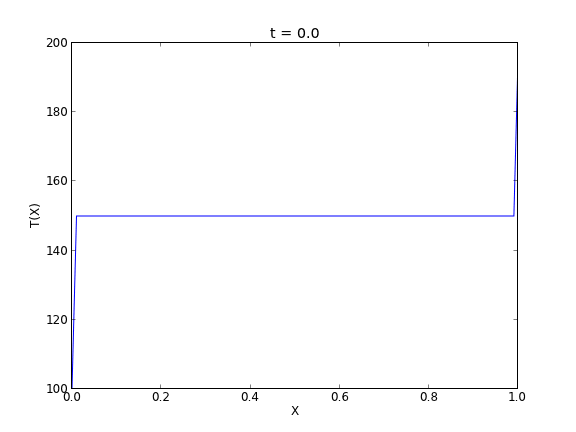Transient heat conduction - partial differential equations

| categories: pde | tags: heat transfer | View Comments

We solved a steady state BVP modeling heat conduction. Today we examine the transient behavior of a rod at constant T put between two heat reservoirs at different temperatures, again T1 = 100, and T2 = 200. The rod will start at 150. Over time, we should expect a solution that approaches the steady state solution: a linear temperature profile from one side of the rod to the other.

$$\frac{\partial u}{\partial t} = k \frac{\partial^2 u}{\partial x^2}$$

at $$t=0$$, in this example we have $$u_0(x) = 150$$ as an initial condition. with boundary conditions $$u(0,t)=100$$ and $$u(L,t)=200$$.

In Matlab there is the pdepe command. There is not yet a PDE solver in scipy. Instead, we will utilze the method of lines to solve this problem. We discretize the rod into segments, and approximate the second derivative in the spatial dimension as $$\frac{\partial^2 u}{\partial x^2} = (u(x + h) - 2 u(x) + u(x-h))/ h^2$$ at each node. This leads to a set of coupled ordinary differential equations that is easy to solve.

Let us say the rod has a length of 1, $$k=0.02$$, and solve for the time-dependent temperature profiles.

import numpy as np
from scipy.integrate import odeint
import matplotlib.pyplot as plt

N = 100  # number of points to discretize
L = 1.0
X = np.linspace(0, L, N) # position along the rod
h = L / (N - 1)

k = 0.02

def odefunc(u, t):
dudt = np.zeros(X.shape)

dudt = 0 # constant at boundary condition
dudt[-1] = 0

# now for the internal nodes
for i in range(1, N-1):
dudt[i] = k * (u[i + 1] - 2*u[i] + u[i - 1]) / h**2

return dudt

init = 150.0 * np.ones(X.shape) # initial temperature
init = 100.0  # one boundary condition
init[-1] = 200.0 # the other boundary condition

tspan = np.linspace(0.0, 5.0, 100)
sol = odeint(odefunc, init, tspan)

for i in range(0, len(tspan), 5):
plt.plot(X, sol[i], label='t={0:1.2f}'.format(tspan[i]))

# put legend outside the figure
plt.legend(loc='center left', bbox_to_anchor=(1, 0.5))
plt.xlabel('X position')
plt.ylabel('Temperature')

# adjust figure edges so the legend is in the figure
plt.savefig('images/pde-transient-heat-1.png')

# Make a 3d figure
from mpl_toolkits.mplot3d import Axes3D
fig = plt.figure()

SX, ST = np.meshgrid(X, tspan)
ax.plot_surface(SX, ST, sol, cmap='jet')
ax.set_xlabel('X')
ax.set_ylabel('time')
ax.set_zlabel('T')
ax.view_init(elev=15, azim=-124) # adjust view so it is easy to see
plt.savefig('images/pde-transient-heat-3d.png')

# animated solution. We will use imagemagick for this

# we save each frame as an image, and use the imagemagick convert command to
# make an animated gif
for i in range(len(tspan)):
plt.clf()
plt.plot(X, sol[i])
plt.xlabel('X')
plt.ylabel('T(X)')
plt.title('t = {0}'.format(tspan[i]))
plt.savefig('___t{0:03d}.png'.format(i))

import commands
print commands.getoutput('convert -quality 100 ___t*.png images/transient_heat.gif')
print commands.getoutput('rm ___t*.png') #remove temp files


This version of the graphical solution is not that easy to read, although with some study you can see the solution evolves from the initial condition which is flat, to the steady state solution which is a linear temperature ramp.The 3d version may be easier to interpret. The temperature profile starts out flat, and gradually changes to the linear ramp.Finally, the animated solution.